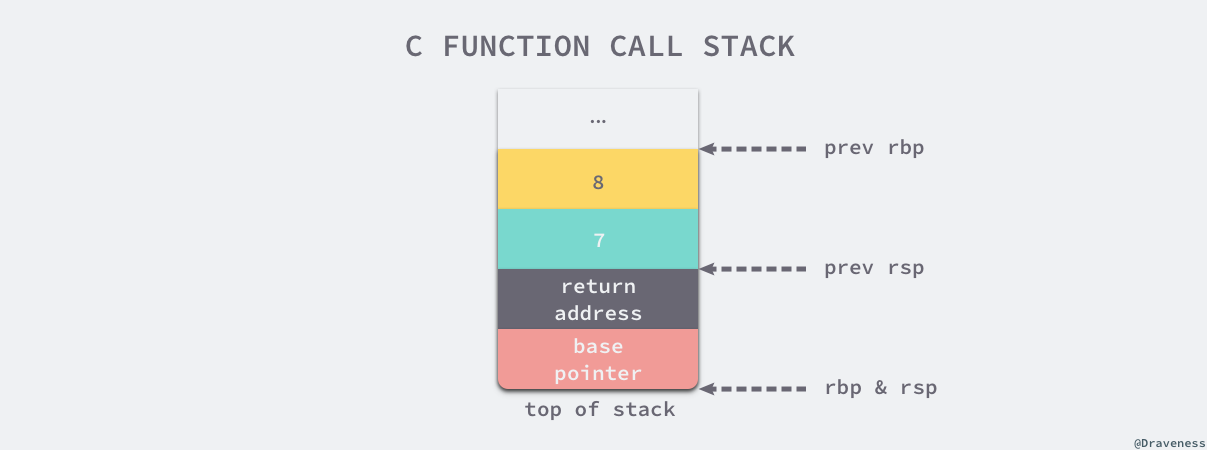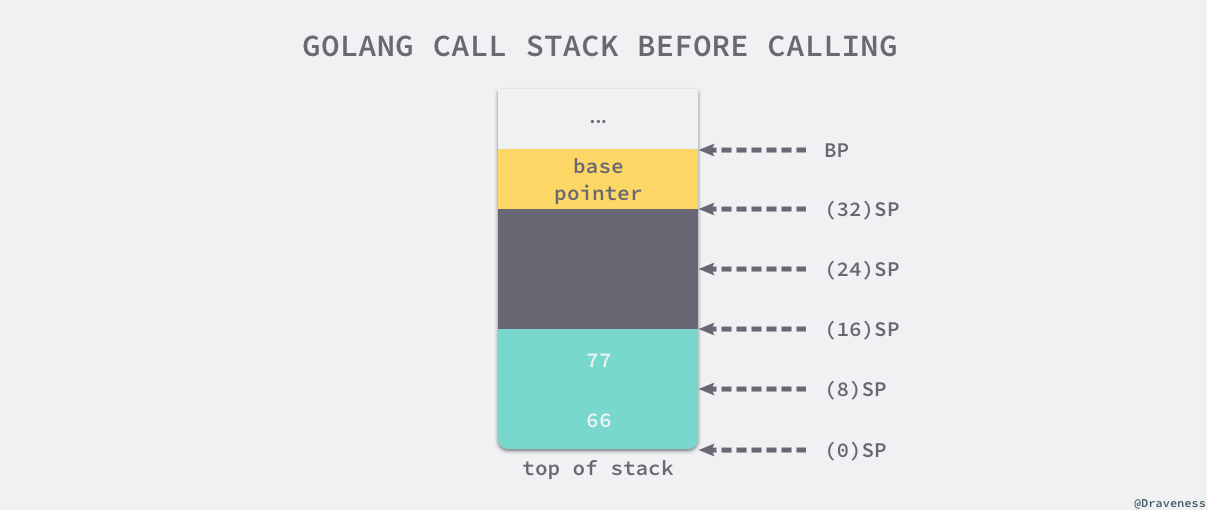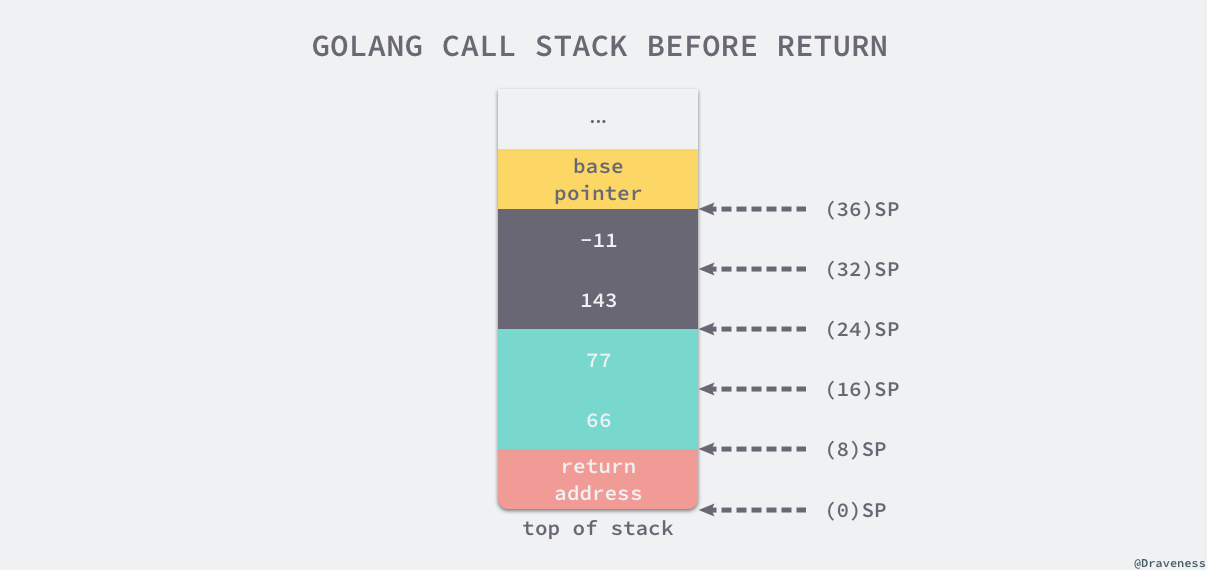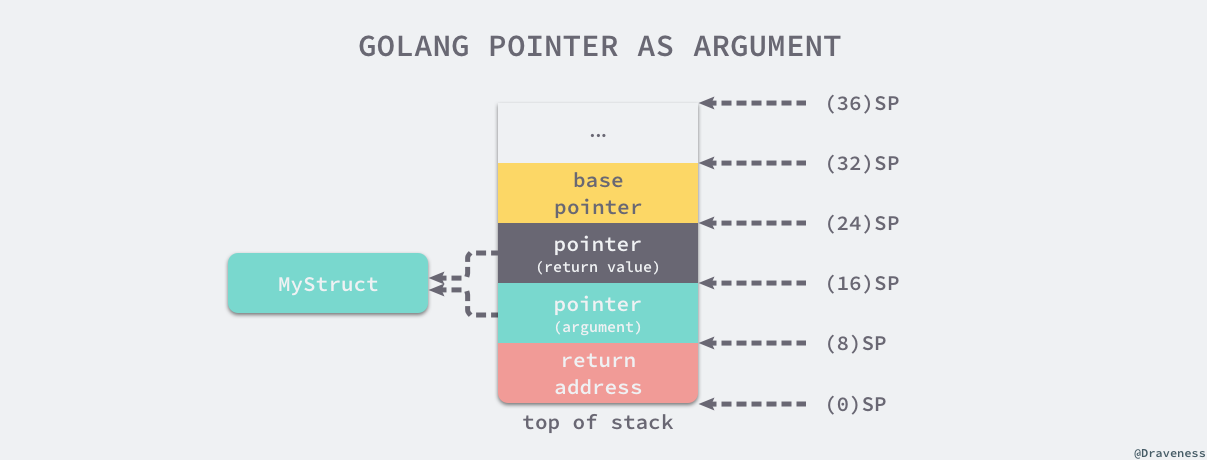# 理解 Golang 中函数调用的原理# 2.1 函数调用

## __ 1. 调用惯例

somefunction(arg0, arg1)

### __ 1.1. C 语言

$gcc --versiongcc (Ubuntu 4.8.2-19ubuntu1) 4.8.2Copyright (C) 2013 Free Software Foundation, Inc.This is free software; see the source for copying conditions. There is NOwarranty; not even for MERCHANTABILITY or FITNESS FOR A PARTICULAR PURPOSE.$ uname -aLinux iZ255w13cy6Z 3.13.0-32-generic #57-Ubuntu SMP Tue Jul 15 03:51:08 UTC 2014 x86_64 x86_64 x86_64 GNU/Linux

gcc 和 clang 虽然在编译 C 语言代码时生成的汇编语言可能有比较大的差别，但是生成代码的结构不会有太大的区别，需要注意的是不同内核版本的操作系统生成的汇编指令可能有比较大的不同，不过对于我们这些只是想要了解实现原理的开发者来说没有太多的影响。

int my_function(int arg1, int arg2) {    return arg1 + arg2;} int main() {    int i = my_function(1, 2);}

my_function:.LFB0:    .cfi_startproc    pushq    %rbp    .cfi_def_cfa_offset 16    .cfi_offset 6, -16    movq    %rsp, %rbp    .cfi_def_cfa_register 6    movl    %edi, -4(%rbp)    movl    %esi, -8(%rbp)    movl    -8(%rbp), %eax    movl    -4(%rbp), %edx    addl    %edx, %eax // arg1 + arg2    popq    %rbp    .cfi_def_cfa 7, 8    ret    .cfi_endprocmain:.LFB1:    .cfi_startproc    pushq    %rbp    .cfi_def_cfa_offset 16    .cfi_offset 6, -16    movq    %rsp, %rbp    .cfi_def_cfa_register 6    subq    $16, %rsp movl$2, %esi // 处理第二个参数    movl    $1, %edi // 处理第一个参数 call my_function movl %eax, -4(%rbp) leave .cfi_def_cfa 7, 8 ret .cfi_endproc 我们可以看到在调用 my_function 函数之前，上述代码将该函数需要的两个参数分别存到了 edi 和 esi 寄存器中；在 my_function 执行时，它会先从寄存器中取出数据并放置到堆栈上，随后通过汇编指令在 eax 寄存器上进行计算，最后的结果其实是通过另一个寄存器 eax 返回的，main 函数在 my_function 返回之后将返回值存储到堆栈上的 i 变量中。 当我们将 my_function 函数的参数增加至 8 个之后，我们再重新编译当前的 C 语言代码，这时可以得到更长的汇编语言代码： 复制代码 // main.cint my_function(int arg1, int arg2, int arg3, int arg4, int arg5, int arg6, int arg7, int arg8) { return arg1 + arg2 + arg3 + arg4 + arg5 + arg6 + arg7 + arg8;} int main() { my_function(1, 2, 3, 4, 5, 6, 7, 8);} // main.smain:.LFB1: .cfi_startproc pushq %rbp .cfi_def_cfa_offset 16 .cfi_offset 6, -16 movq %rsp, %rbp .cfi_def_cfa_register 6 subq$16, %rsp    movl    $8, 8(%rsp) movl$7, (%rsp)    movl    $6, %r9d movl$5, %r8d    movl    $4, %ecx movl$3, %edx    movl    $2, %esi movl$1, %edi    call    my_function    leave    .cfi_def_cfa 7, 8    ret    .cfi_endprocmain 函数中，通过 SUBQ $40, SP 指令一共在栈上分配了 40 字节的内存空间，最开始的 8 个字节存储了 main 函数的栈基址指针，之后的 16 个字节是为函数 myFunction 的两个返回值预留的空间，最后的 16 字节存储了该函数调用时需要的两个参数，压栈的顺序和 C 语言中一样，也是从右到左。 接下来就调用了汇编指令 CALL "".myFunction(SB)，这个指令首先会将当前函数 main 的返回值压栈，然后改变当前的栈指针 SP 并开始执行 myFunction 的汇编指令： 复制代码 "".myFunction STEXT nosplit size=49 args=0x20 locals=0x0 0x0000 00000 (main.go:3) TEXT "".myFunction(SB), NOSPLIT,$0-32    0x0000 00000 (main.go:3)    MOVQ    $0, "".~r2+24(SP) // 初始化第一个返回值 0x0009 00009 (main.go:3) MOVQ$0, "".~r3+32(SP) // 初始化第二个返回值    0x0012 00018 (main.go:4)    MOVQ    "".a+8(SP), AX    // AX = 66    0x0017 00023 (main.go:4)    ADDQ    "".b+16(SP), AX   // AX = AX + 77 = 143    0x001c 00028 (main.go:4)    MOVQ    AX, "".~r2+24(SP) // (24)SP = AX = 143    0x0021 00033 (main.go:4)    MOVQ    "".a+8(SP), AX    // AX = 66    0x0026 00038 (main.go:4)    SUBQ    "".b+16(SP), AX   // AX = AX - 77 = -11    0x002b 00043 (main.go:4)    MOVQ    AX, "".~r3+32(SP) // (32)SP = AX = -11    0x0030 00048 (main.go:4)    RETmyFunction 函数返回之后，main 函数就会通过以下的指令来恢复栈基址指针并销毁已经失去作用的 40 字节的栈空间：

func myFunction(i int, arr int) {    i = 29    arr = 88    fmt.Printf("in my_funciton - i=(%d, %p) arr=(%v, %p)\n", i, &i, arr, &arr)} func main() {    i := 30    arr := int{66, 77}    fmt.Printf("before calling - i=(%d, %p) arr=(%v, %p)\n", i, &i, arr, &arr)    myFunction(i, arr)    fmt.Printf("after  calling - i=(%d, %p) arr=(%v, %p)\n", i, &i, arr, &arr)} $go run main.gobefore calling - i=(30, 0xc000072008) arr=([66 77], 0xc000072010)in my_funciton - i=(29, 0xc000072028) arr=([66 88], 0xc000072040)after calling - i=(30, 0xc000072008) arr=([66 77], 0xc000072010) 可以看到在 myFunction 中对参数的修改也仅仅影响了当前的函数栈，同时并没有对调用方的函数栈有任何的影响，我们也能给出如下的结论 - Go 语言中对于整型和数组类型的参数都是值传递的，也就是在调用函数时会对内容进行拷贝，需要注意的是如果当前数组的大小非常的大，这种直接复制传值的方式就会对性能造成比较大的影响。 ### __ 2.2. 结构体和指针 接下来我们需要再来看一下在 Golang 中的另外两种常见的类型 - 结构体和指针在参数的传递过程中是传值还是传引用的。在这里定义一个只包含一个成员变量的简单结构体 MyStruct 以及接受两个参数的 myFunction 方法： 复制代码 type MyStruct struct { i int} func myFunction(a MyStruct, b *MyStruct) { a.i = 31 b.i = 41 fmt.Printf("in my_function - a=(%d, %p) b=(%v, %p)\n", a, &a, b, &b)} func main() { a := MyStruct{i: 30} b := &MyStruct{i: 40} fmt.Printf("before calling - a=(%d, %p) b=(%v, %p)\n", a, &a, b, &b) myFunction(a, b) fmt.Printf("after calling - a=(%d, %p) b=(%v, %p)\n", a, &a, b, &b)}$ go run main.gobefore calling - a=({30}, 0xc000018178) b=(&{40}, 0xc00000c028)in my_function - a=({31}, 0xc000018198) b=(&{41}, 0xc00000c038)after calling  - a=({30}, 0xc000018178) b=(&{41}, 0xc00000c028)

package main import "unsafe"import "fmt" type MyStruct struct {    i int    j int} func myFunction(ms *MyStruct) {    ptr := unsafe.Pointer(ms)    for i := 0; i < 2; i++ {        c := (*int)(unsafe.Pointer((uintptr(ptr) + uintptr(8*i))))        *c += i + 1        fmt.Printf("[%p] %d\n", c, *c)    }} func main() {    a := &MyStruct{i: 40, j: 50}    myFunction(a)    fmt.Printf("[%p] %v\n", a, a)} $go run main.go[0xc000018180] 41[0xc000018188] 52[0xc000018180] &{41 52} 在这段代码中，我们直接通过指针的方式修改结构体中的成员变量，结构体在内存中其实就是一篇连续的空间，其中的多个成员变量连续布局，指向结构体的指针其实也是指向这个结构体的首地址，如果我们将 MyStruct 指针修改成 int 类型的，那么对新指针的去引用（dereference）就会返回一个整型变量 i，由于一个 int 类型的变量占 8 个字节，所以将指针移动 8 位之后就能获取下一个结构的成员变量 j 如果我们将上述代码中大部分无关的信息省略并只对其中的 myFuncionMyStruct 这两段代码进行编译： 复制代码 type MyStruct struct { i int j int} func myFunction(ms *MyStruct) *MyStruct { return ms} // assembly"".myFunction STEXT nosplit size=20 args=0x10 locals=0x0 0x0000 00000 (main.go:8) TEXT "".myFunction(SB), NOSPLIT,$0-16    0x0000 00000 (main.go:8)    FUNCDATA    $0, gclocals·aef1f7ba6e2630c93a51843d99f5a28a(SB) 0x0000 00000 (main.go:8) FUNCDATA$1, gclocals·33cdeccccebe80329f1fdbee7f5874cb(SB)    0x0000 00000 (main.go:8)    MOVQ    \$0, "".~r1+16(SP) // 初始化返回值    0x0009 00009 (main.go:9)    MOVQ    "".ms+8(SP), AX   // 复制引用    0x000e 00014 (main.go:9)    MOVQ    AX, "".~r1+16(SP) // 返回引用    0x0013 00019 (main.go:9)    RET## __ 3. 总结

1. 通过堆栈传递参数，入栈的顺序是从右到左；
2. 函数返回值通过堆栈传递并由调用者预先分配内存空间；
3. 调用函数时都是传值，接收方会对入参进行复制再计算；

## __ 4. Reference

** 本文转载自 Draveness 技术博客。